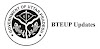### Applied Mathematics 1st Semester Topics

Lists Of Chapter And Their Topics In Applied Mathematics 1st Semester Up Polytechnic

#### 1.2 APPLIED MATHEMATICS – I

• Binomial theorem to evaluate mathematical problems
• Determinants properties and Crammer’s rule to evaluate mathematical problems
• Dot & Cross product of vectors to illustrate the mathematical problems
• Use of complex numbers in various mathematical problems
• Differential calculus and higher order to evaluate mathematical problems
• Find velocity, acceleration, errors and approximation in mathematical illustration with application of derivatives.

### DETAILED CONTENTS -Applied Mathematics 1 PDF Free Download Up Polytechnic

1. Algebra -I

1.1 Series : AP Series and GP Series; And Sum, nth term, Mean

1.2 Binomial theorem in AP and GP for positive, negative and fractional index (without proof). Execution of Binomial theorem.

1.3 Determinants: Determinant of order 2 and 3 Of Elementary properties, Multiplication of algebraic equation, Crammer’s rule, Consistency of equation.

2. Algebra- II

2.1 Vector Algebra: Dot Product And Cross Product, Scalar Product & Vector Triple Product.

2.2 Complex number: Representation, Modulus and amplitude Demoivre theorem, its application in solving algebraic equations, Complex numbers, Mod. Function and its properties..

3. Trigonometry

3.1 Relating the difference between sides and angles of a triangle: Statement of various formulae which shows relationship between sides and angle of a triangle.

3.2 Inverse circular functions : Simple case only

4. Differential Calculus – I

4.1 Functions and their graphs, range and domain, elementary methods of finding limits (right and left), Functions, limits, continuity, elementary test for continuity and differentiability.

4.2 Trigonometric functions, exponential function, Logarithmic differentiation, Differentiation of Inverse trigonometric function, Methods of finding derivative, Function of a function, Differentiation of implicit functions.

5. Differential Calculus – II

5.1 Higher order derivatives, Leibnitz theorem (without proof). Simple applications.

5.2 Application – Finding Tangents, Normal, Points of Maxima/Minima, Increasing/Decreasing functions, Rate, Measure, velocity, Acceleration, Errors and approximation.

Previous article
Next article

### Trending Posts